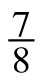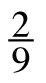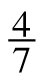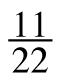### Home > CC2 > Chapter 2 > Lesson 2.2.2 > Problem2-54

2-54.

Rewrite the following fractions as decimals and decide if they are terminating or repeating decimals. Show your work and explain how you made your decision. Homework Help ✎

1.Divide $7$ by $8$. Do you get a repeating decimal?

Since $7$ divided by $8$ is $0.875$, and this does not repeat without end, it is a terminating decimal.

1.Here is Kelly's work.

$\ \ \ \ 0.22\\9\longdiv{2.00}\\\ \ \ \ 1.8\\\ \ \ \ \ \ .20\\\ \ \ \ \ \ .18\\\ \ \ \ \ \ldots\\\ \ \ \ \ \ldots$

Does it look like the decimal will repeat?

1.See parts (a) and (b).

Repeating.

1.See parts (a) and (b).